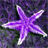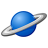# QlikView App Dev

Discussion Board for collaboration related to QlikView App Development.

Announcements
Join us for a live Q&A! September 21, 10 AM ET - Onboarding Fast in Qlik Sense SaaS! REGISTER
cancel
Showing results for
Did you mean:Creator II

## count pass/fail students in pivot table

this is live example for count pass/fail students..

i need to count the failed students in pivot table like the example

regards.

13 RepliesCreator II

Hi,

Can you please explain what you want exactly in pivot . Which count ?

Thanks,

BunnyCreator III

use this Expression in pivot table

count({<Result_field={"fail"}>}Result_field )Creator II
Author

Dear Ahmar,

i think it's wrong to use only pass_fail field = fail ?! because i need to count the students which failed in 1 unit only, and 2 units, and 3 units.. so i need something like this:

count(aggr(-(count({<[Pass_Fail]={'f'}>} People_ID) =2), People_ID))

but this expression doesn't give me actual result !

Regards.Not applicable

!

Hi,

Please see file attached for a solution i have altered your formula to

Failed 1 Unit = sum(if((Aggr(sum(if(PASS_FAIL = 'F',1,0)), People_ID))=1,1,0))

Failed 2 Unit = sum(if((Aggr(sum(if(PASS_FAIL = 'F',1,0)), People_ID))=2,1,0))

Failed 3 Unit = sum(if((Aggr(sum(if(PASS_FAIL = 'F',1,0)), People_ID))=3,1,0))  .... etc .

I would recomend that you change your script to add a new field that does the count on the back end.

Use Script Below:

SQL SELECT "DEGREE" as "Degree",

"EVENT_LONG_NAME" as "Event",

"CURRICULUM" as "Curriculum",

"CLASS_LEVEL" as "Class_Level",

"PEOPLE_ID" as "People_ID",

FROM "PCDB_PROD20150906".dbo."VW_TotalRegistration";

"EVENT_ID",

"PASS_FAIL",

"PEOPLE_ID" as "People_ID"

FROM "PCDB_PROD20150906".dbo."VW_Pass/Fail";

Left Join

People_ID,

Count(if("PASS_FAIL"= 'F', 1, 0)) as Sub_Failed

Group By People_ID;

Regards

RahulCreator II
Author

Thanks a lot for helpcount(DISTINCT{<Pass_Fail = {'F'}>} People_ID)

can you please send me the right expression because this one is wrong

Regard.Creator II
Author

and for the first query you give it to me, when i reload the data in qlikview this error is appear:

Script Error:

Field not fount - <PASS_FAIL>

left Join

People_IDm

Count(if("PASS_FAIL" ='F',1,0)) as Sub_Failed

Group By People_IDNot applicable

try

Count(if(PASS_FAIL ='F',PASS_FAIL ,0)) as Sub_FailedNot applicable

try

count(DISTINCT if(PASS_FAIL = 'F', People_ID)) or

count(DISTINCT{<PASS_FAIL = {'F'}>} People_ID)Creator II

Hi,

Try this,

sum(if((Aggr(sum(if(PASS_FAIL = 'F',1,0)), People_ID))=1,1,0))+

sum(if((Aggr(sum(if(PASS_FAIL = 'F',1,0)), People_ID))=2,1,0))+

sum(if((Aggr(sum(if(PASS_FAIL = 'F',1,0)), People_ID))=3,1,0))+

sum(if((Aggr(sum(if(PASS_FAIL = 'F',1,0)), People_ID))=4,1,0))

Hope including all failed  is the desired value .

Thanks,

BunnyCommunity Browser